Updating search results...

# 81 Results

View
Selected filters:
• cl2lesson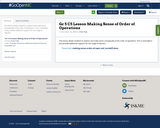Conditional Remix & Share Permitted
CC BY-NC-SA
Rating
0.0 stars

This lesson allows students to explore and make sense conceptually of the order of operations. This is remixable if you provide additional supports for the range of learners.

Subject:
Mathematics
Material Type:
Activity/Lab
Lesson Plan
11/16/2019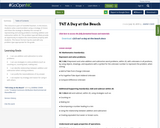Conditional Remix & Share Permitted
CC BY-NC-SA
Rating
0.0 stars

This resource is part of Tools4NCTeachers.

In this lesson, students solve a word problem using a strategy of choice and share the strategy to develop the concept of representing and solving problems involving addition and subtraction within 20. This problem type will likely provide an opportunity to explore the commutative property with students. This lesson format may be used with any problem type appropriate for the grade.

Subject:
Mathematics
Material Type:
Lesson
Lesson Plan
11/06/2019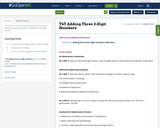Conditional Remix & Share Permitted
CC BY-NC-SA
Rating
0.0 stars

This resource is part of Tools4NCTeachers.

In this lesson, students explore approaches for adding three two-digit numbers.

Subject:
Mathematics
Material Type:
Lesson
Lesson Plan
11/06/2019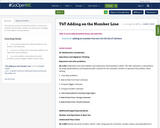Conditional Remix & Share Permitted
CC BY-NC-SA
Rating
0.0 stars

This resource is part of Tools4NCTeachers.

In this lesson students use the open number line and various strategies to solve addition story problems.

Subject:
Mathematics
Material Type:
Lesson
Lesson Plan
11/06/2019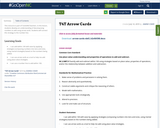Conditional Remix & Share Permitted
CC BY-NC-SA
Rating
0.0 stars

This resource is part of Tools4NCTeachers.

In this lesson, students develop an understanding of decomposing numbers to add using arrow cards. Students will connect this strategy to the number line.

Subject:
Mathematics
Material Type:
Lesson
Lesson Plan
11/06/2019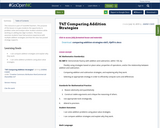Conditional Remix & Share Permitted
CC BY-NC-SA
Rating
0.0 stars

This resource is part of Tools4NCTeachers.

This purpose of this lesson is to give students the opportunity to problem solve and analyze other student solutions while working on adding two-digit numbers. This lesson assumes students have had previous experience with multiple addition strategies and that the class has posted strategy supports.

Subject:
Mathematics
Material Type:
Lesson
Lesson Plan
11/06/2019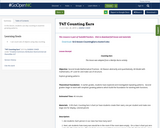Conditional Remix & Share Permitted
CC BY-NC-SA
Rating
0.0 stars

In this lesson, students use skip counting to examine growing patterns.

Subject:
Mathematics
Material Type:
Lesson
Lesson Plan
11/06/2019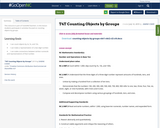Conditional Remix & Share Permitted
CC BY-NC-SA
Rating
0.0 stars

This resource is part of Tools4NCTeachers.

In this lesson students participate in activities focused on counting objects by groups.

Subject:
Mathematics
Material Type:
Lesson
Lesson Plan
11/06/2019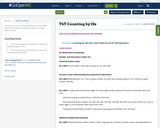Conditional Remix & Share Permitted
CC BY-NC-SA
Rating
0.0 stars

This resource is part of Tools4NCTeachers.

In this lesson students participate in activities focused on counting objects by tens.

Subject:
Mathematics
Material Type:
Lesson
Lesson Plan
11/06/2019Conditional Remix & Share Permitted
CC BY-NC-SA
Rating
0.0 stars

This resource is part of Tools4NCTeachers.

In this activity, students are given clues about a mystery number card. Students listen to the clues, find the corresponding quantity, and then identify the matching numeral. The goal of this activity is to develop flexibility in representing numbers in a variety of ways.

Subject:
Mathematics
Material Type:
Lesson
Lesson Plan
11/06/2019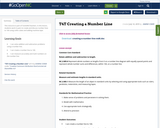Conditional Remix & Share Permitted
CC BY-NC-SA
Rating
0.0 stars

This resource is part of Tools4NCTeachers.

In this lesson, students work in pairs to create and discuss number lines to 100 using unifix cubes and adding machine tape.

Subject:
Mathematics
Material Type:
Lesson
Lesson Plan
11/06/2019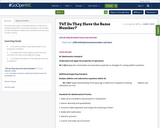Conditional Remix & Share Permitted
CC BY-NC-SA
Rating
0.0 stars

This resource is part of Tools4NCTeachers.

In this lesson, students explore the communitive property in the context of a word problem to determine if students have the same number of items

Subject:
Mathematics
Material Type:
Lesson
Lesson Plan
11/06/2019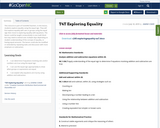Conditional Remix & Share Permitted
CC BY-NC-SA
Rating
0.0 stars

This resource is part of Tools4NCTeachers.

In this lesson, students explore equality using the equal sign. They will first explore equality with sets or groups using the equal sign then move to exploring equality with equations. The lesson could be taught consecutively in one math block but may need to extend over multiple days depending on student understanding of the concept of equality, or having equal amounts. The lesson could also be modified or extended by repeating tasks and discussion with more emphasis on subtraction.

Subject:
Mathematics
Material Type:
Lesson
Lesson Plan
11/06/2019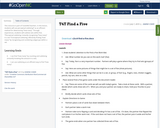Conditional Remix & Share Permitted
CC BY-NC-SA
Rating
0.0 stars

This resource is part of Tools4NCTeachers.

In this lesson, students will use perceptual subitizing* as an efficient method for determining "how many". Through experiences, students will subitize sets within five.

*Perceptual subitizing: instantly recognizing "how many" in a set
*Conceptual subitizing: efficiently finding "how many" by recognizing and combining subgroups within a set.

Subject:
Mathematics
Material Type:
Lesson
Lesson Plan
11/06/2019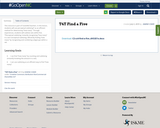Conditional Remix & Share Permitted
CC BY-NC-SA
Rating
0.0 stars

This resource is part of Tools4NCTeachers.

In this lesson, students will use perceptual subitizing* as an efficient method for determining "how many". Through experiences, students will subitize sets within five.

*Perceptual subitizing: instantly recognizing "how many" in a set
Conceptual subitizing: efficiently finding "how many" by recognizing and combining subgroups within a set.

Subject:
Mathematics
Material Type:
Lesson
Lesson Plan
11/06/2019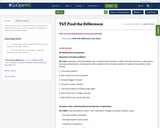Conditional Remix & Share Permitted
CC BY-NC-SA
Rating
0.0 stars

This resource is part of Tools4NCTeachers.

In this lesson students use the open number and various strategies to solve two-step story problems.

Subject:
Mathematics
Material Type:
Lesson
Lesson Plan
11/06/2019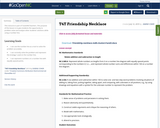Conditional Remix & Share Permitted
CC BY-NC-SA
Rating
0.0 stars

This resource is part of Tools4NCTeachers.

This purpose of this lesson is to give students the opportunity to problem solve and analyze other students' solutions while using a number line.

Subject:
Mathematics
Material Type:
Lesson
Lesson Plan
11/06/2019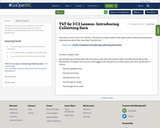Conditional Remix & Share Permitted
CC BY-NC-SA
Rating
0.0 stars

This lesson is from Tools 4 NC Teachers. This lesson provides students with opportunities to become introduced to collecting data about their classmates’ favorite food.

Subject:
Mathematics
Material Type:
Activity/Lab
Lesson Plan
11/06/2019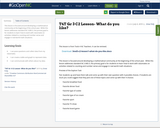Conditional Remix & Share Permitted
CC BY-NC-SA
Rating
0.0 stars

This lesson is focused around developing a mathematical community at the beginning of the school year. While this lesson addresses standard NC.3.MD.3, the primary goal is for students to learn how to work with classmates on activities related to counting and number sense and engage in real-world math situations.

Subject:
Mathematics
Material Type:
Activity/Lab
Lesson Plan
11/06/2019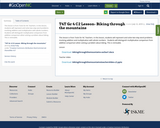Conditional Remix & Share Permitted
CC BY-NC-SA
Rating
0.0 stars

This lesson is from Tools for NC Teachers. In this lesson, students will represent and solve two-step word problems involving addition and multiplication with whole numbers. Students will distinguish multiplicative comparison from additive comparison when solving a problem about biking. This is remixable.

Subject:
Mathematics
Material Type:
Activity/Lab
Lesson Plan